# HashMap、ConcurrentHashMap原理分析

2018/08/05 11:17

Java7和Java8的HashMap差别还是比较大的，源码角度来看也越来越复杂，本文将依次从Java7的HashMap以及Java8的HashMap，Java7的ConcurrentHashMap和Java8的ConcurrentHashMap的顺序来逐步分析源码，深入理解他们的原理。

Hashmap的key和value允许存放null值

## Java7 HashMap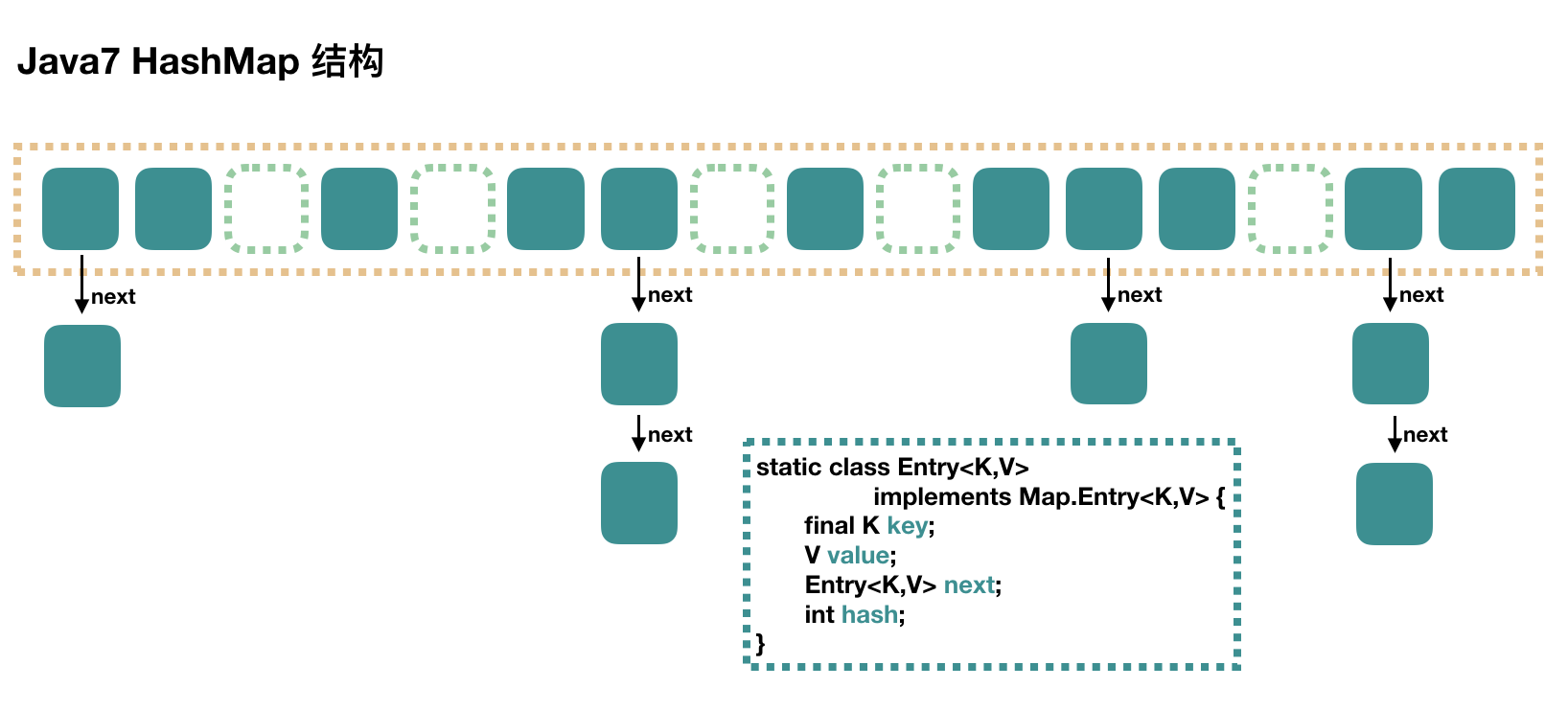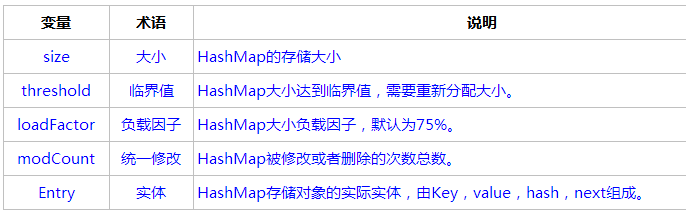size的大小始终为2的n次方，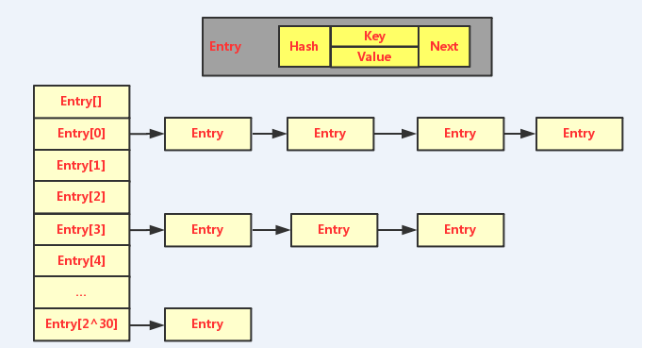### put 过程分析

put的过程比较简单，跟着代码看一遍就能看懂

public V put(K key, V value) {
// 当插入第一个元素的时候，需要先初始化数组大小
if (table == EMPTY_TABLE) {
inflateTable(threshold);
}
// 如果 key 为 null，感兴趣的可以往里看，最终会将这个 entry 放到 table 中
if (key == null)
return putForNullKey(value);
// 1. 求 key 的 hash 值
int hash = hash(key);
// 2. 找到对应的数组下标
int i = indexFor(hash, table.length);
// 3. 遍历一下对应下标处的链表，看是否有重复的 key 已经存在，
//    如果有，直接覆盖，put 方法返回旧值就结束了
for (Entry<K,V> e = table[i]; e != null; e = e.next) {
Object k;
if (e.hash == hash && ((k = e.key) == key || key.equals(k))) {
V oldValue = e.value;
e.value = value;
e.recordAccess(this);
return oldValue;
}
}

modCount++;
// 4. 不存在重复的 key，将此 entry 添加到链表中，细节后面说
return null;
}

#### 数组初始化

private void inflateTable(int toSize) {
// 保证数组大小一定是 2 的 n 次方。
// 比如这样初始化：new HashMap(20)，那么处理成初始数组大小是 32
int capacity = roundUpToPowerOf2(toSize);
threshold = (int) Math.min(capacity * loadFactor, MAXIMUM_CAPACITY + 1);
// 算是初始化数组吧
table = new Entry[capacity];
initHashSeedAsNeeded(capacity); //ignore
}

#### 计算具体数组位置

static int indexFor(int hash, int length) {
// assert Integer.bitCount(length) == 1 : "length must be a non-zero power of 2";
return hash & (length-1);
}

#### 添加节点到链表中

void addEntry(int hash, K key, V value, int bucketIndex) {
// 如果当前 HashMap 大小已经达到了阈值，并且新值要插入的数组位置已经有元素了，那么要扩容
if ((size >= threshold) && (null != table[bucketIndex])) {
// 扩容，后面会介绍一下
resize(2 * table.length);
// 扩容以后，重新计算 hash 值
hash = (null != key) ? hash(key) : 0;
// 重新计算扩容后的新的下标
bucketIndex = indexFor(hash, table.length);
}
// 往下看
createEntry(hash, key, value, bucketIndex);
}
// 这个很简单，其实就是将新值放到链表的表头，然后 size++
void createEntry(int hash, K key, V value, int bucketIndex) {
Entry<K,V> e = table[bucketIndex];
table[bucketIndex] = new Entry<>(hash, key, value, e);
size++;
}

#### 数组扩容

void resize(int newCapacity) {
Entry[] oldTable = table;
int oldCapacity = oldTable.length;
if (oldCapacity == MAXIMUM_CAPACITY) {
threshold = Integer.MAX_VALUE;
return;
}
// 新的数组
Entry[] newTable = new Entry[newCapacity];
// 将原来数组中的值迁移到新的更大的数组中
transfer(newTable, initHashSeedAsNeeded(newCapacity));
table = newTable;
threshold = (int)Math.min(newCapacity * loadFactor, MAXIMUM_CAPACITY + 1);

void transfer(Entry[] newTable, boolean rehash) {
int newCapacity = newTable.length;
for (Entry<K,V> e : table) {
while(null != e) {
Entry<K,V> next = e.next;
if (rehash) {
e.hash = null == e.key ? 0 : hash(e.key);
}
int i = indexFor(e.hash, newCapacity);
e.next = newTable[i];
newTable[i] = e;
e = next;
}
}
}

1. 对索引数组中的元素遍历
2. 对链表上的每一个节点遍历：用 next 取得要转移那个元素的下一个，将 e 转移到新 Hash 表的头部，使用头插法插入节点。
3. 循环2，直到链表节点全部转移
4. 循环1，直到所有索引数组全部转移

### get 过程分析

1. 根据 key 计算 hash 值。
2. 找到相应的数组下标：hash & (length – 1)。
3. 遍历该数组位置处的链表，直到找到相等(==或equals)的 key。
public V get(Object key) {
// 之前说过，key 为 null 的话，会被放到 table，所以只要遍历下 table 处的链表就可以了
if (key == null)
return getForNullKey();
//
Entry<K,V> entry = getEntry(key);

return null == entry ? null : entry.getValue();

getEntry(key):

final Entry<K,V> getEntry(Object key) {
if (size == 0) {
return null;
}

int hash = (key == null) ? 0 : hash(key);
// 确定数组下标，然后从头开始遍历链表，直到找到为止
for (Entry<K,V> e = table[indexFor(hash, table.length)];
e != null;
e = e.next) {
Object k;
if (e.hash == hash &&
((k = e.key) == key || (key != null && key.equals(k))))
return e;
}
return null;
}

## Java8 HashMap

Java8 对 HashMap 进行了一些修改，最大的不同就是利用了红黑树，所以其由 数组+链表+红黑树 组成。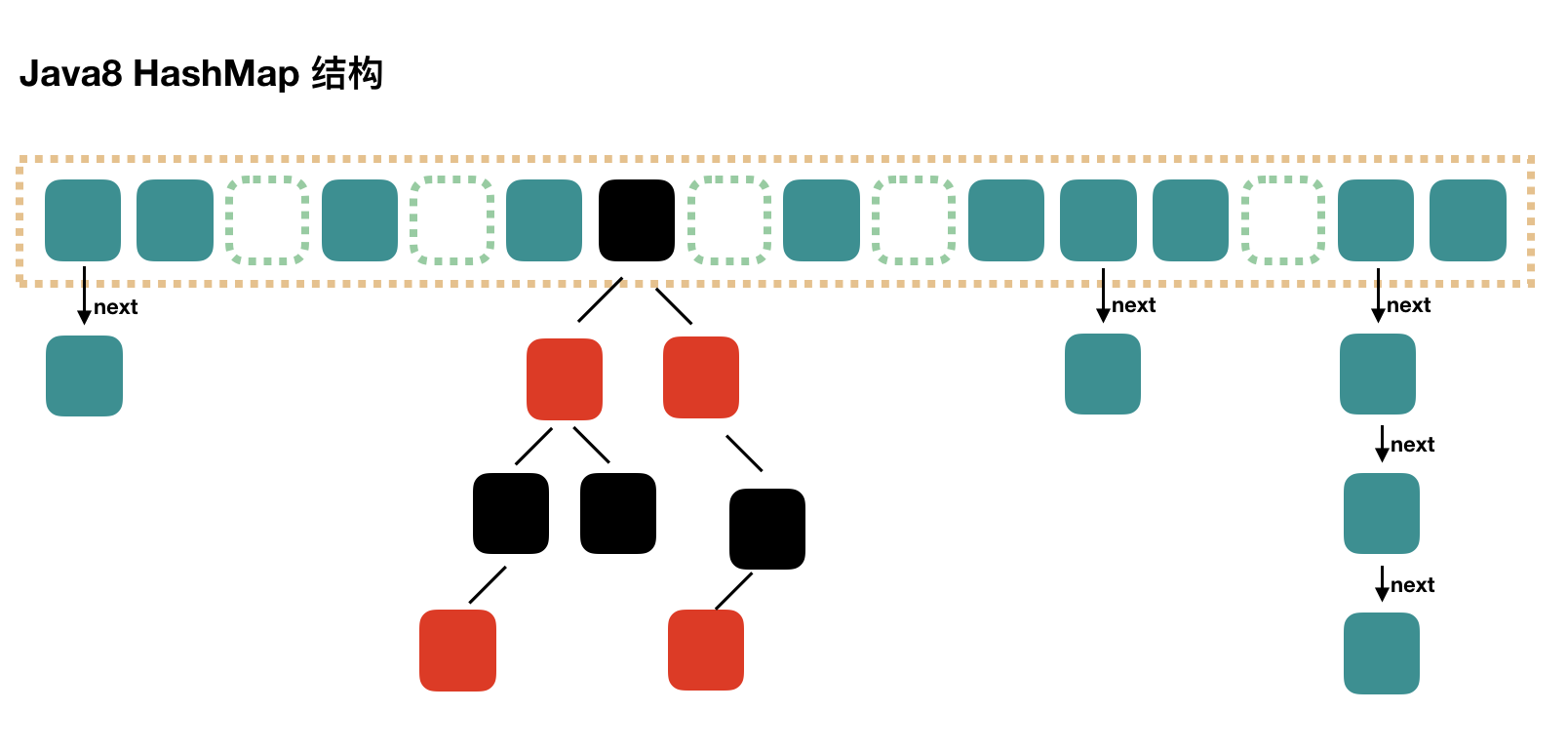Java7 中使用 Entry 来代表每个 HashMap 中的数据节点，Java8 中使用 Node，基本没有区别，都是 key，value，hash 和 next 这四个属性，不过，Node 只能用于链表的情况，红黑树的情况需要使用 TreeNode。

### put 过程分析

public V put(K key, V value) {
return putVal(hash(key), key, value, false, true);
}

// 第三个参数 onlyIfAbsent 如果是 true，那么只有在不存在该 key 时才会进行 put 操作
// 第四个参数 evict 我们这里不关心
final V putVal(int hash, K key, V value, boolean onlyIfAbsent,
boolean evict) {
Node<K,V>[] tab; Node<K,V> p; int n, i;
// 第一次 put 值的时候，会触发下面的 resize()，类似 java7 的第一次 put 也要初始化数组长度
// 第一次 resize 和后续的扩容有些不一样，因为这次是数组从 null 初始化到默认的 16 或自定义的初始容量
if ((tab = table) == null || (n = tab.length) == 0)
n = (tab = resize()).length;
// 找到具体的数组下标，如果此位置没有值，那么直接初始化一下 Node 并放置在这个位置就可以了
if ((p = tab[i = (n - 1) & hash]) == null)
tab[i] = newNode(hash, key, value, null);

else {// 数组该位置有数据
Node<K,V> e; K k;
// 首先，判断该位置的第一个数据和我们要插入的数据，key 是不是"相等"，如果是，取出这个节点
if (p.hash == hash &&
((k = p.key) == key || (key != null && key.equals(k))))
e = p;
// 如果该节点是代表红黑树的节点，调用红黑树的插值方法，本文不展开说红黑树
else if (p instanceof TreeNode)
e = ((TreeNode<K,V>)p).putTreeVal(this, tab, hash, key, value);
else {
// 到这里，说明数组该位置上是一个链表
for (int binCount = 0; ; ++binCount) {
// 插入到链表的最后面(Java7 是插入到链表的最前面)
if ((e = p.next) == null) {
p.next = newNode(hash, key, value, null);
// TREEIFY_THRESHOLD 为 8，所以，如果新插入的值是链表中的第 9 个
// 会触发下面的 treeifyBin，也就是将链表转换为红黑树
if (binCount >= TREEIFY_THRESHOLD - 1) // -1 for 1st
treeifyBin(tab, hash);
break;
}
// 如果在该链表中找到了"相等"的 key(== 或 equals)
if (e.hash == hash &&
((k = e.key) == key || (key != null && key.equals(k))))
// 此时 break，那么 e 为链表中[与要插入的新值的 key "相等"]的 node
break;
p = e;
}
}
// e!=null 说明存在旧值的key与要插入的key"相等"
// 对于我们分析的put操作，下面这个 if 其实就是进行 "值覆盖"，然后返回旧值
if (e != null) {
V oldValue = e.value;
if (!onlyIfAbsent || oldValue == null)
e.value = value;
afterNodeAccess(e);
return oldValue;
}
}
++modCount;
// 如果 HashMap 由于新插入这个值导致 size 已经超过了阈值，需要进行扩容
if (++size > threshold)
resize();
afterNodeInsertion(evict);
return null;
}

#### 数组扩容

resize() 方法用于初始化数组或数组扩容，每次扩容后，容量为原来的 2 倍，并进行数据迁移。

 1 final Node<K,V>[] resize() {
2     Node<K,V>[] oldTab = table;
3     int oldCap = (oldTab == null) ? 0 : oldTab.length;
4     int oldThr = threshold;
5     int newCap, newThr = 0;
6     if (oldCap > 0) { // 对应数组扩容
7         if (oldCap >= MAXIMUM_CAPACITY) {
8             threshold = Integer.MAX_VALUE;
9             return oldTab;
10         }
11         // 将数组大小扩大一倍
12         else if ((newCap = oldCap << 1) < MAXIMUM_CAPACITY &&
13                  oldCap >= DEFAULT_INITIAL_CAPACITY)
14             // 将阈值扩大一倍
15             newThr = oldThr << 1; // double threshold
16     }
17     else if (oldThr > 0) // 对应使用 new HashMap(int initialCapacity) 初始化后，第一次 put 的时候
18         newCap = oldThr;
19     else {// 对应使用 new HashMap() 初始化后，第一次 put 的时候
20         newCap = DEFAULT_INITIAL_CAPACITY;
21         newThr = (int)(DEFAULT_LOAD_FACTOR * DEFAULT_INITIAL_CAPACITY);
22     }
23
24     if (newThr == 0) {
25         float ft = (float)newCap * loadFactor;
26         newThr = (newCap < MAXIMUM_CAPACITY && ft < (float)MAXIMUM_CAPACITY ?
27                   (int)ft : Integer.MAX_VALUE);
28     }
29     threshold = newThr;
30
31     // 用新的数组大小初始化新的数组
32     Node<K,V>[] newTab = (Node<K,V>[])new Node[newCap];
33     table = newTab; // 如果是初始化数组，到这里就结束了，返回 newTab 即可
34
35     if (oldTab != null) {
36         // 开始遍历原数组，进行数据迁移。
37         for (int j = 0; j < oldCap; ++j) {
38             Node<K,V> e;
39             if ((e = oldTab[j]) != null) {
40                 oldTab[j] = null;
41                 // 如果该数组位置上只有单个元素，那就简单了，简单迁移这个元素就可以了
42                 if (e.next == null)
43                     newTab[e.hash & (newCap - 1)] = e;
44                 // 如果是红黑树，具体我们就不展开了
45                 else if (e instanceof TreeNode)
46                     ((TreeNode<K,V>)e).split(this, newTab, j, oldCap);
47                 else {
48                     // 这块是处理链表的情况，
49                     // 需要将此链表拆成两个链表，放到新的数组中，并且保留原来的先后顺序
51                     Node<K,V> loHead = null, loTail = null;
52                     Node<K,V> hiHead = null, hiTail = null;
53                     Node<K,V> next;
54                     do {
55                         next = e.next;
56                         if ((e.hash & oldCap) == 0) {
57                             if (loTail == null)
59                             else
60                                 loTail.next = e;
61                             loTail = e;
62                         }
63                         else {
64                             if (hiTail == null)
66                             else
67                                 hiTail.next = e;
68                             hiTail = e;
69                         }
70                     } while ((e = next) != null);
71                     if (loTail != null) {
72                         loTail.next = null;
73                         // 第一条链表
75                     }
76                     if (hiTail != null) {
77                         hiTail.next = null;
78                         // 第二条链表的新的位置是 j + oldCap，这个很好理解
79                         newTab[j + oldCap] = hiHead;
80                     }
81                 }
82             }
83         }
84     }
85     return newTab;
86 }

### get 过程分析

1. 计算 key 的 hash 值，根据 hash 值找到对应数组下标: hash & (length-1)
2. 判断数组该位置处的元素是否刚好就是我们要找的，如果不是，走第三步
3. 判断该元素类型是否是 TreeNode，如果是，用红黑树的方法取数据，如果不是，走第四步
4. 遍历链表，直到找到相等(==或equals)的 key
public V get(Object key) {
Node<K,V> e;
return (e = getNode(hash(key), key)) == null ? null : e.value;
}

final Node<K,V> getNode(int hash, Object key) {
Node<K,V>[] tab; Node<K,V> first, e; int n; K k;
if ((tab = table) != null && (n = tab.length) > 0 &&
(first = tab[(n - 1) & hash]) != null) {
// 判断第一个节点是不是就是需要的
if (first.hash == hash && // always check first node
((k = first.key) == key || (key != null && key.equals(k))))
return first;
if ((e = first.next) != null) {
// 判断是否是红黑树
if (first instanceof TreeNode)
return ((TreeNode<K,V>)first).getTreeNode(hash, key);

// 链表遍历
do {
if (e.hash == hash &&
((k = e.key) == key || (key != null && key.equals(k))))
return e;
} while ((e = e.next) != null);
}
}
return null;
}

## HashMap为什么不是线程安全的

HashMap众所周知是线程不安全的，所有方法没采用同步处理，无法做到线程同步（线程安全）也是可以想象得到的。HashMap的线程不安全性主要体现在以下三个方面：

1.在hashmap做put操作的时候会调用下面方法：

// 新增Entry。将“key-value”插入指定位置，bucketIndex是位置索引。
void addEntry(int hash, K key, V value, int bucketIndex) {
// 保存“bucketIndex”位置的值到“e”中
Entry<K,V> e = table[bucketIndex];
// 设置“bucketIndex”位置的元素为“新Entry”，
// 设置“e”为“新Entry的下一个节点”
table[bucketIndex] = new Entry<K,V>(hash, key, value, e);
// 若HashMap的实际大小 不小于 “阈值”，则调整HashMap的大小
if (size++ >= threshold)
resize(2 * table.length);
}

2.删除键值对的代码

final Entry<K,V> removeEntryForKey(Object key) {
// 获取哈希值。若key为null，则哈希值为0；否则调用hash()进行计算
int hash = (key == null) ? 0 : hash(key.hashCode());
int i = indexFor(hash, table.length);
Entry<K,V> prev = table[i];
Entry<K,V> e = prev;

// 删除链表中“键为key”的元素
// 本质是“删除单向链表中的节点”
while (e != null) {
Entry<K,V> next = e.next;
Object k;
if (e.hash == hash &&
((k = e.key) == key || (key != null && key.equals(k)))) {
modCount++;
size--;
if (prev == e)
table[i] = next;
else
prev.next = next;
e.recordRemoval(this);
return e;
}
prev = e;
e = next;
}      

## Java7 ConcurrentHashMap

ConcurrentHashMap 和 HashMap 思路是差不多的，但是因为它支持并发操作，所以要复杂一些。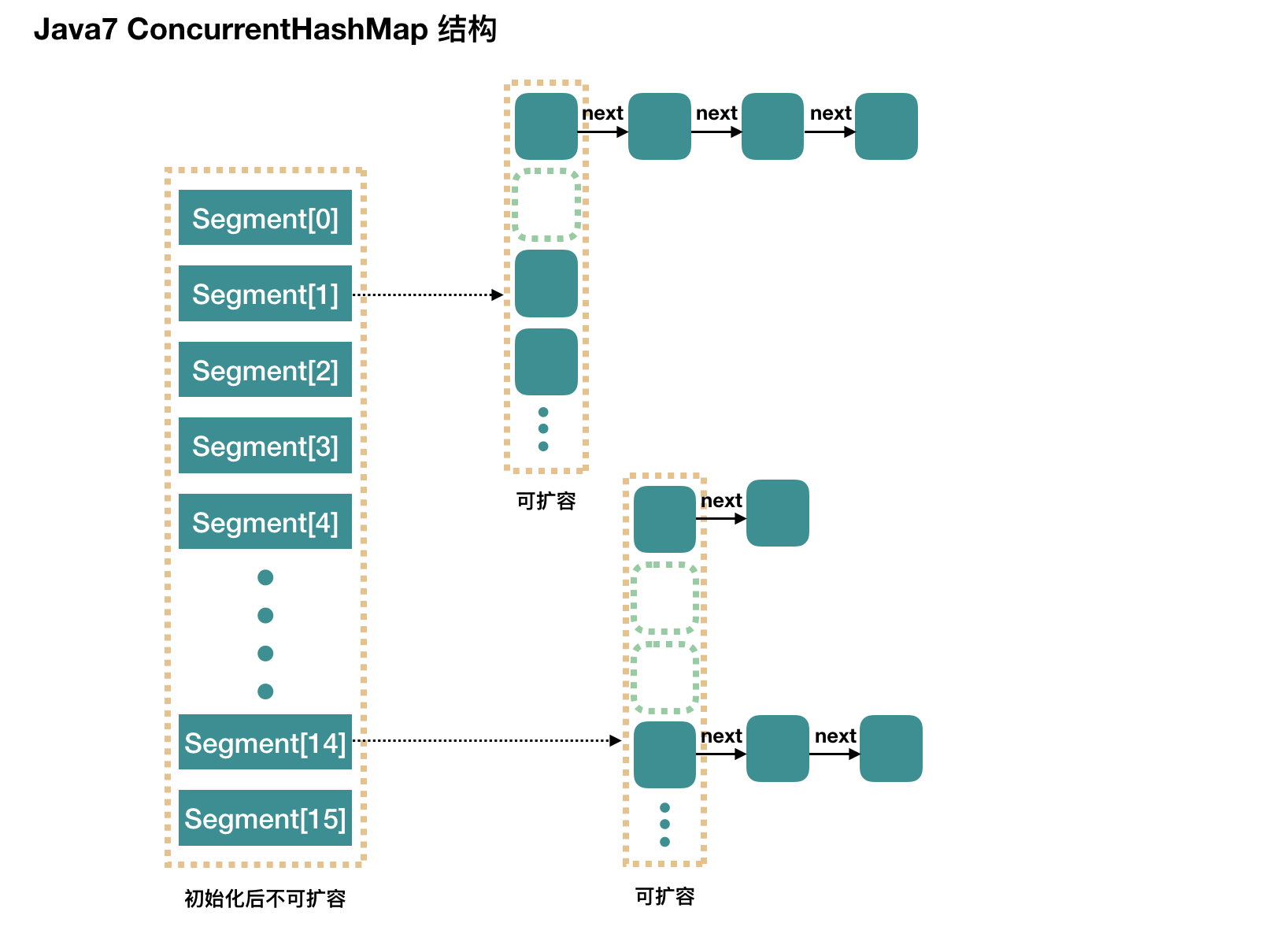concurrencyLevel：并行级别、并发数、Segment 数，怎么翻译不重要，理解它。默认是 16，也就是说 ConcurrentHashMap 有 16 个 Segments，所以理论上，这个时候，最多可以同时支持 16 个线程并发写，只要它们的操作分别分布在不同的 Segment 上。这个值可以在初始化的时候设置为其他值，但是一旦初始化以后，它是不可以扩容的。

### 初始化

initialCapacity：初始容量，这个值指的是整个 ConcurrentHashMap 的初始容量，实际操作的时候需要平均分给每个 Segment。

public ConcurrentHashMap(int initialCapacity,
if (!(loadFactor > 0) || initialCapacity < 0 || concurrencyLevel <= 0)
throw new IllegalArgumentException();
if (concurrencyLevel > MAX_SEGMENTS)
concurrencyLevel = MAX_SEGMENTS;
// Find power-of-two sizes best matching arguments
int sshift = 0;
int ssize = 1;
// 计算并行级别 ssize，因为要保持并行级别是 2 的 n 次方
while (ssize < concurrencyLevel) {
++sshift;
ssize <<= 1;
}
// 我们这里先不要那么烧脑，用默认值，concurrencyLevel 为 16，sshift 为 4
// 那么计算出 segmentShift 为 28，segmentMask 为 15，后面会用到这两个值
this.segmentShift = 32 - sshift;

if (initialCapacity > MAXIMUM_CAPACITY)
initialCapacity = MAXIMUM_CAPACITY;

// initialCapacity 是设置整个 map 初始的大小，
// 这里根据 initialCapacity 计算 Segment 数组中每个位置可以分到的大小
// 如 initialCapacity 为 64，那么每个 Segment 或称之为"槽"可以分到 4 个
int c = initialCapacity / ssize;
if (c * ssize < initialCapacity)
++c;
// 默认 MIN_SEGMENT_TABLE_CAPACITY 是 2，这个值也是有讲究的，因为这样的话，对于具体的槽上，
// 插入一个元素不至于扩容，插入第二个的时候才会扩容
int cap = MIN_SEGMENT_TABLE_CAPACITY;
while (cap < c)
cap <<= 1;

// 创建 Segment 数组，
// 并创建数组的第一个元素 segment
Segment<K,V> s0 =
(HashEntry<K,V>[])new HashEntry[cap]);
Segment<K,V>[] ss = (Segment<K,V>[])new Segment[ssize];
// 往数组写入 segment
UNSAFE.putOrderedObject(ss, SBASE, s0); // ordered write of segments
this.segments = ss;
}

• Segment 数组长度为 16，不可以扩容
• Segment[i] 的默认大小为 2，负载因子是 0.75，得出初始阈值为 1.5，也就是以后插入第一个元素不会触发扩容，插入第二个会进行第一次扩容
• 这里初始化了 segment，其他位置还是 null，至于为什么要初始化 segment，后面的代码会介绍
• 当前 segmentShift 的值为 32 – 4 = 28，segmentMask 为 16 – 1 = 15，姑且把它们简单翻译为移位数和掩码，这两个值马上就会用到

### put 过程分析

public V put(K key, V value) {
Segment<K,V> s;
if (value == null)
throw new NullPointerException();
// 1. 计算 key 的 hash 值
int hash = hash(key);
// 2. 根据 hash 值找到 Segment 数组中的位置 j
//    hash 是 32 位，无符号右移 segmentShift(28) 位，剩下低 4 位，
//    然后和 segmentMask(15) 做一次与操作，也就是说 j 是 hash 值的最后 4 位，也就是槽的数组下标
int j = (hash >>> segmentShift) & segmentMask;
// 刚刚说了，初始化的时候初始化了 segment，但是其他位置还是 null，
// ensureSegment(j) 对 segment[j] 进行初始化
if ((s = (Segment<K,V>)UNSAFE.getObject          // nonvolatile; recheck
(segments, (j << SSHIFT) + SBASE)) == null) //  in ensureSegment
s = ensureSegment(j);
// 3. 插入新值到 槽 s 中
return s.put(key, hash, value, false);
}

Segment 内部是由 数组+链表 组成的。

final V put(K key, int hash, V value, boolean onlyIfAbsent) {
// 在往该 segment 写入前，需要先获取该 segment 的独占锁
//    先看主流程，后面还会具体介绍这部分内容
HashEntry<K,V> node = tryLock() ? null :
scanAndLockForPut(key, hash, value);
V oldValue;
try {
// 这个是 segment 内部的数组
HashEntry<K,V>[] tab = table;
// 再利用 hash 值，求应该放置的数组下标
int index = (tab.length - 1) & hash;
// first 是数组该位置处的链表的表头
HashEntry<K,V> first = entryAt(tab, index);

// 下面这串 for 循环虽然很长，不过也很好理解，想想该位置没有任何元素和已经存在一个链表这两种情况
for (HashEntry<K,V> e = first;;) {
if (e != null) {
K k;
if ((k = e.key) == key ||
(e.hash == hash && key.equals(k))) {
oldValue = e.value;
if (!onlyIfAbsent) {
// 覆盖旧值
e.value = value;
++modCount;
}
break;
}
// 继续顺着链表走
e = e.next;
}
else {
// node 到底是不是 null，这个要看获取锁的过程，不过和这里都没有关系。
// 如果不为 null，那就直接将它设置为链表表头；如果是null，初始化并设置为链表表头。
if (node != null)
node.setNext(first);
else
node = new HashEntry<K,V>(hash, key, value, first);

int c = count + 1;
// 如果超过了该 segment 的阈值，这个 segment 需要扩容
if (c > threshold && tab.length < MAXIMUM_CAPACITY)
rehash(node); // 扩容后面也会具体分析
else
// 没有达到阈值，将 node 放到数组 tab 的 index 位置，
// 其实就是将新的节点设置成原链表的表头
setEntryAt(tab, index, node);
++modCount;
count = c;
oldValue = null;
break;
}
}
} finally {
// 解锁
unlock();
}
return oldValue;
}

#### 获取写入锁: scanAndLockForPut

private HashEntry<K,V> scanAndLockForPut(K key, int hash, V value) {
HashEntry<K,V> first = entryForHash(this, hash);
HashEntry<K,V> e = first;
HashEntry<K,V> node = null;
int retries = -1; // negative while locating node

// 循环获取锁
while (!tryLock()) {
HashEntry<K,V> f; // to recheck first below
if (retries < 0) {
if (e == null) {
if (node == null) // speculatively create node
// 进到这里说明数组该位置的链表是空的，没有任何元素
// 当然，进到这里的另一个原因是 tryLock() 失败，所以该槽存在并发，不一定是该位置
node = new HashEntry<K,V>(hash, key, value, null);
retries = 0;
}
else if (key.equals(e.key))
retries = 0;
else
// 顺着链表往下走
e = e.next;
}
// 重试次数如果超过 MAX_SCAN_RETRIES（单核1多核64），那么不抢了，进入到阻塞队列等待锁
//    lock() 是阻塞方法，直到获取锁后返回
else if (++retries > MAX_SCAN_RETRIES) {
lock();
break;
}
else if ((retries & 1) == 0 &&
// 这个时候是有大问题了，那就是有新的元素进到了链表，成为了新的表头
//     所以这边的策略是，相当于重新走一遍这个 scanAndLockForPut 方法
(f = entryForHash(this, hash)) != first) {
e = first = f; // re-traverse if entry changed
retries = -1;
}
}
return node;
}

#### 初始化槽: ensureSegment

ConcurrentHashMap 初始化的时候会初始化第一个槽 segment，对于其他槽来说，在插入第一个值的时候进行初始化。

private Segment<K,V> ensureSegment(int k) {
final Segment<K,V>[] ss = this.segments;
long u = (k << SSHIFT) + SBASE; // raw offset
Segment<K,V> seg;
if ((seg = (Segment<K,V>)UNSAFE.getObjectVolatile(ss, u)) == null) {
// 这里看到为什么之前要初始化 segment 了，
// 使用当前 segment 处的数组长度和负载因子来初始化 segment[k]
// 为什么要用“当前”，因为 segment 可能早就扩容过了
Segment<K,V> proto = ss;
int cap = proto.table.length;
int threshold = (int)(cap * lf);

// 初始化 segment[k] 内部的数组
HashEntry<K,V>[] tab = (HashEntry<K,V>[])new HashEntry[cap];
if ((seg = (Segment<K,V>)UNSAFE.getObjectVolatile(ss, u))
== null) { // 再次检查一遍该槽是否被其他线程初始化了。

Segment<K,V> s = new Segment<K,V>(lf, threshold, tab);
// 使用 while 循环，内部用 CAS，当前线程成功设值或其他线程成功设值后，退出
while ((seg = (Segment<K,V>)UNSAFE.getObjectVolatile(ss, u))
== null) {
if (UNSAFE.compareAndSwapObject(ss, u, null, seg = s))
break;
}
}
}
return seg;
}


#### 扩容: rehash

// 方法参数上的 node 是这次扩容后，需要添加到新的数组中的数据。
private void rehash(HashEntry<K,V> node) {
HashEntry<K,V>[] oldTable = table;
int oldCapacity = oldTable.length;
// 2 倍
int newCapacity = oldCapacity << 1;
// 创建新数组
HashEntry<K,V>[] newTable =
(HashEntry<K,V>[]) new HashEntry[newCapacity];
// 新的掩码，如从 16 扩容到 32，那么 sizeMask 为 31，对应二进制 ‘000...00011111’
int sizeMask = newCapacity - 1;

// 遍历原数组，老套路，将原数组位置 i 处的链表拆分到 新数组位置 i 和 i+oldCap 两个位置
for (int i = 0; i < oldCapacity ; i++) {
// e 是链表的第一个元素
HashEntry<K,V> e = oldTable[i];
if (e != null) {
HashEntry<K,V> next = e.next;
// 计算应该放置在新数组中的位置，
// 假设原数组长度为 16，e 在 oldTable 处，那么 idx 只可能是 3 或者是 3 + 16 = 19
int idx = e.hash & sizeMask;
if (next == null)   // 该位置处只有一个元素，那比较好办
newTable[idx] = e;
else { // Reuse consecutive sequence at same slot
// e 是链表表头
HashEntry<K,V> lastRun = e;
// idx 是当前链表的头结点 e 的新位置
int lastIdx = idx;

// 下面这个 for 循环会找到一个 lastRun 节点，这个节点之后的所有元素是将要放到一起的
for (HashEntry<K,V> last = next;
last != null;
last = last.next) {
int k = last.hash & sizeMask;
if (k != lastIdx) {
lastIdx = k;
lastRun = last;
}
}
// 将 lastRun 及其之后的所有节点组成的这个链表放到 lastIdx 这个位置
newTable[lastIdx] = lastRun;
// 下面的操作是处理 lastRun 之前的节点，
//    这些节点可能分配在另一个链表中，也可能分配到上面的那个链表中
for (HashEntry<K,V> p = e; p != lastRun; p = p.next) {
V v = p.value;
int h = p.hash;
int k = h & sizeMask;
HashEntry<K,V> n = newTable[k];
newTable[k] = new HashEntry<K,V>(h, p.key, v, n);
}
}
}
}
// 将新来的 node 放到新数组中刚刚的 两个链表之一 的 头部
node.setNext(newTable[nodeIndex]);
newTable[nodeIndex] = node;
table = newTable;
}

### get 过程分析

1. 计算 key 的 hash 值，根据 hash 值找到对应数组下标: hash & (length-1)
2. 判断数组该位置处的元素是否刚好就是我们要找的，如果不是，走第三步
3. 判断该元素类型是否是 TreeNode，如果是，用红黑树的方法取数据，如果不是，走第四步
4. 遍历链表，直到找到相等(==或equals)的 key
public V get(Object key) {
Node<K,V> e;
return (e = getNode(hash(key), key)) == null ? null : e.value;
}

final Node<K,V> getNode(int hash, Object key) {
Node<K,V>[] tab; Node<K,V> first, e; int n; K k;
if ((tab = table) != null && (n = tab.length) > 0 &&
(first = tab[(n - 1) & hash]) != null) {
// 判断第一个节点是不是就是需要的
if (first.hash == hash && // always check first node
((k = first.key) == key || (key != null && key.equals(k))))
return first;
if ((e = first.next) != null) {
// 判断是否是红黑树
if (first instanceof TreeNode)
return ((TreeNode<K,V>)first).getTreeNode(hash, key);

// 链表遍历
do {
if (e.hash == hash &&
((k = e.key) == key || (key != null && key.equals(k))))
return e;
} while ((e = e.next) != null);
}
}
return null;
}

## Java8 ConcurrentHashMap

Java 8 CouncurrentHashMap的结构和Java 8 HashMap很像，不过要保证线程安全，源码上要复杂很多。Java 8的ConcurrentHashMap取消segments字段，直接采用transient volatile HashEntry<K,V> table保存数据，采用table数组元素作为锁，从而实现了对每一行数据进行加锁，进一步减少并发冲突的概率。

Node节点的value和next都用volatile修饰，保证并发性。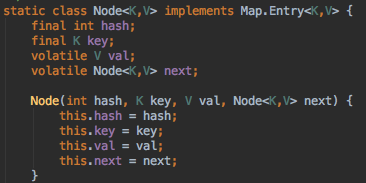### 初始化

// 这构造函数里，什么都不干
public ConcurrentHashMap() {
}
public ConcurrentHashMap(int initialCapacity) {
if (initialCapacity < 0)
throw new IllegalArgumentException();
int cap = ((initialCapacity >= (MAXIMUM_CAPACITY >>> 1)) ?
MAXIMUM_CAPACITY :
tableSizeFor(initialCapacity + (initialCapacity >>> 1) + 1));
this.sizeCtl = cap;
}

### put 过程分析

public V put(K key, V value) {
return putVal(key, value, false);
}
final V putVal(K key, V value, boolean onlyIfAbsent) {
if (key == null || value == null) throw new NullPointerException();
// 得到 hash 值
// 用于记录相应链表的长度
int binCount = 0;
for (Node<K,V>[] tab = table;;) {
Node<K,V> f; int n, i, fh;
// 如果数组"空"，进行数组初始化
if (tab == null || (n = tab.length) == 0)
// 初始化数组，后面会详细介绍
tab = initTable();

// 找该 hash 值对应的数组下标，得到第一个节点 f
else if ((f = tabAt(tab, i = (n - 1) & hash)) == null) {
// 如果数组该位置为空，
//    用一次 CAS 操作将这个新值放入其中即可，这个 put 操作差不多就结束了，可以拉到最后面了
//          如果 CAS 失败，那就是有并发操作，进到下一个循环就好了
if (casTabAt(tab, i, null,
new Node<K,V>(hash, key, value, null)))
break;                   // no lock when adding to empty bin
}
// hash 居然可以等于 MOVED，这个需要到后面才能看明白，不过从名字上也能猜到，肯定是因为在扩容
else if ((fh = f.hash) == MOVED)
// 帮助数据迁移，这个等到看完数据迁移部分的介绍后，再理解这个就很简单了
tab = helpTransfer(tab, f);

else { // 到这里就是说，f 是该位置的头结点，而且不为空

V oldVal = null;
// 获取数组该位置的头结点的监视器锁
synchronized (f) {
if (tabAt(tab, i) == f) {
if (fh >= 0) { // 头结点的 hash 值大于 0，说明是链表
// 用于累加，记录链表的长度
binCount = 1;
// 遍历链表
for (Node<K,V> e = f;; ++binCount) {
K ek;
// 如果发现了"相等"的 key，判断是否要进行值覆盖，然后也就可以 break 了
if (e.hash == hash &&
((ek = e.key) == key ||
(ek != null && key.equals(ek)))) {
oldVal = e.val;
if (!onlyIfAbsent)
e.val = value;
break;
}
// 到了链表的最末端，将这个新值放到链表的最后面
Node<K,V> pred = e;
if ((e = e.next) == null) {
pred.next = new Node<K,V>(hash, key,
value, null);
break;
}
}
}
else if (f instanceof TreeBin) { // 红黑树
Node<K,V> p;
binCount = 2;
// 调用红黑树的插值方法插入新节点
if ((p = ((TreeBin<K,V>)f).putTreeVal(hash, key,
value)) != null) {
oldVal = p.val;
if (!onlyIfAbsent)
p.val = value;
}
}
}
}
// binCount != 0 说明上面在做链表操作
if (binCount != 0) {
// 判断是否要将链表转换为红黑树，临界值和 HashMap 一样，也是 8
if (binCount >= TREEIFY_THRESHOLD)
// 这个方法和 HashMap 中稍微有一点点不同，那就是它不是一定会进行红黑树转换，
// 如果当前数组的长度小于 64，那么会选择进行数组扩容，而不是转换为红黑树
//    具体源码我们就不看了，扩容部分后面说
treeifyBin(tab, i);
if (oldVal != null)
return oldVal;
break;
}
}
}
//
return null;
}

### get 过程分析

1. 计算 hash 值
2. 根据 hash 值找到数组对应位置: (n – 1) & h
3. 根据该位置处结点性质进行相应查找
• 如果该位置为 null，那么直接返回 null 就可以了
• 如果该位置处的节点刚好就是我们需要的，返回该节点的值即可
• 如果该位置节点的 hash 值小于 0，说明正在扩容，或者是红黑树，后面我们再介绍 find 方法
• 如果以上 3 条都不满足，那就是链表，进行遍历比对即可
public V get(Object key) {
Node<K,V>[] tab; Node<K,V> e, p; int n, eh; K ek;
if ((tab = table) != null && (n = tab.length) > 0 &&
(e = tabAt(tab, (n - 1) & h)) != null) {
// 判断头结点是否就是我们需要的节点
if ((eh = e.hash) == h) {
if ((ek = e.key) == key || (ek != null && key.equals(ek)))
return e.val;
}
// 如果头结点的 hash 小于 0，说明 正在扩容，或者该位置是红黑树
else if (eh < 0)
// 参考 ForwardingNode.find(int h, Object k) 和 TreeBin.find(int h, Object k)
return (p = e.find(h, key)) != null ? p.val : null;

// 遍历链表
while ((e = e.next) != null) {
if (e.hash == h &&
((ek = e.key) == key || (ek != null && key.equals(ek))))
return e.val;
}
}
return null;
}

## 参考链接：

http://www.importnew.com/28263.html

https://www.cnblogs.com/lchzls/p/6714689.html

https://blog.csdn.net/fjse51/article/details/55260493

0
0 收藏

### 作者的其它热门文章0 评论
0 收藏
0